# Dominoes in Unicode

I was spelunking around in Unicode and found that there are assigned characters for representing domino tiles and that the characters are enumerated in a convenient order. Here is the code chart.

There are codes for representing tiles horizontally or vertically. And even though, for example, the 5-3 is the same domino as the 3-5, there are separate characters for representing the orientation of the tile: one for 3 on the left and one for 5 on the left.

When you include orientation like this, a domino becomes essentially a base 7 number: the number of spots on one end is the number of 7s and the number of spots on the other end is the number of 1s. And the order of the characters corresponds to the order as base 7 numbers:

0-0, 0-1, 0-2, …, 1-0, 1-1, 1-2, … 6-6.

The horizontal dominoes start with the double blank at U+1F031 and the vertical dominoes start with U+1F063, a difference of 32 in hexadecimal or 50 in base 10. So you can rotate a domino tile by adding or subtracting 50 to its code point.

The following tiny Python function gives the codepoint for the domino with a spots on the left (or top) and b spots on the right (or bottom).

```    def code(a, b, wide):
cp = 0x1f031 if wide else 0x1f063
return cp + 7*a + b
```

We can use this function to print a (3, 5) tile horizontally and a (6, 4) tile vertically.

```    print( chr(code(3, 5, True )),
chr(code(6, 4, False)) )
```

To my surprise, my computer had the fonts installed to display the results. This isn’t guaranteed for such high Unicode values.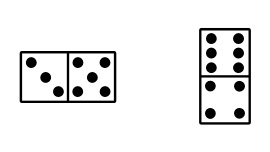# Raging against the machine

Ran across a great quote from Liv Boeree recently:

The problem with raging against the machine is that the machine has learned to feed off rage.

Someone appropriately replied with a screenshot from an episode of Star Trek TOS, Day of the Dove, about a being that feeds off anger, like contemporary media.# Field of order 9

This post will give a detailed example of working in a field with nine elements. This is important because finite fields are not often treated concretely except for the case of prime order.

In my first post on Costas arrays I mentioned in a footnote that Lempel’s algorithm works more generally over any finite field, but I stuck to integers modulo primes to make things simpler.

In this post we’ll create a 7 × 7 Costas array by working in a field with 9 elements. Along the way we’ll explain in great detail how finite field arithmetic works, using the field with 9 elements as an example.

# Costas arrays in Mathematica

A couple days ago I wrote about Costas arrays. In a nutshell, a Costas array of size n is a solution to the n rooks problem, with the added constraint that if you added wires between the rooks, no two wires would have the same length and slope. See the earlier post for more details.

The earlier post implemented the Lempel algorithm in Python. Here we’ll implement it in Mathematica. Lempel’s algorithm says to start with parameters p and a where p is a prime and a is a primitive root mod p . Then you can create a Costas array of size n = p-2 by filling in the (i, j) square if and only if

ai + aj = 1 mod p.

We can implement this in Mathematica by

```    fill[i_, j_, p_, a_] := If[Mod[a^i + a^j, p] == 1, 1, 0]
m[p_, a_] := Table[fill[i, j, p, a], {i, 1, p - 2}, {j, 1, p - 2}]
```

We could visualize the Costas array generated by p = 11 and a = 2 using `ArrayPlot`. The code

`    ArrayPlot[m[11, 2], Mesh -> All]`

Generates the following image.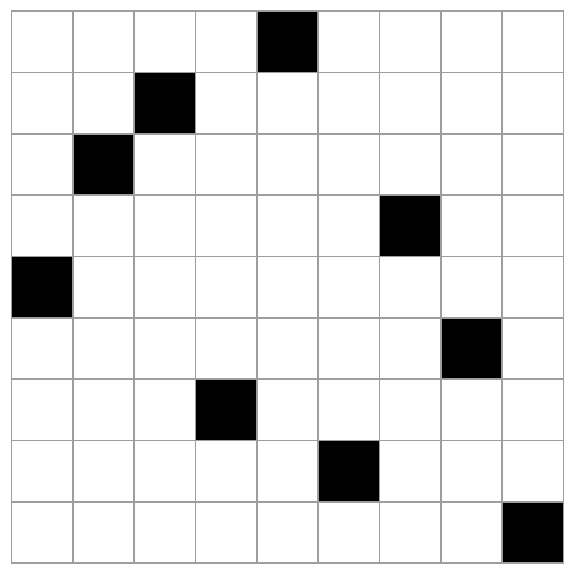Note that the image is symmetric with respect to the main diagonal. That’s because our algorithm is symmetric in i and j. But Costas arrays are not always symmetrical, so this underscores the point that there is no known scalable algorithm for finding all Costas arrays.

In this example we started with knowing that 2 was a primitive root mod 11. We could have had Mathematica pick a primitive root mod p for us by calling `PrimitiveRoot[p]`

Just out of curiosity, let’s redo the example above, but instead of testing whether

ai + aj = 1 mod p.

we’ll just use ai + aj mod p.

The code

`    ArrayPlot[Table[Mod[2^i + 2^j, 11], {i, 1, 9}, {j, 1, 9}]]`

produces the following image.Lempel’s algorithm generalizes to finite fields of any order, not just integers modulo a prime.

# Two-letter vs Three-letter Country Abbreviations

The ISO 3166-1 standard defines three codes for each country: a 2-letter abbreviation, a 3-letter abbreviation, and a 3-digit code.

The 2-letter abbreviations may be familiar because it is very often (but not always ) also the country code top-level domain (ccTLD). For example, AU is the ISO abbreviation for Australia, and .au is the ccTLD.

I was curious about the relation between the two-letter and three-letter abbreviations. Sometimes the former is a prefix of the latter, such as US and USA. Sometimes the latter adds a letter in the middle, such as going from CN to CHN. How common are these two patterns?

I wrote a script using the iso3166 Python module to find out.

Turns out that the prefix pattern is most common, and occurs 63% of the time.

The infix pattern is the next most common, occurring 29% of the time.

Suffixes are rare. There are only for instances where the 2-letter name is the last two letters of the 3-letter name: ATF, MYT, SPM, and SGS.

The remaining possibilities are miscellaneous relations, such as IL and ISR for Israel.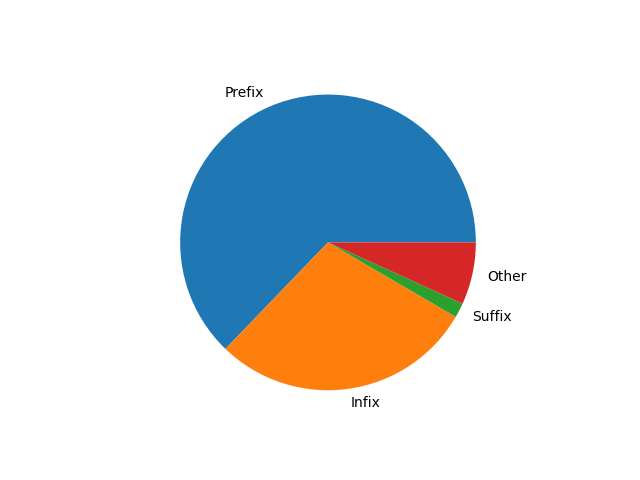Here’s a table of countries and ISO codes in plain text (org-mode) format.

 There are four ccTLDs that are not ISO 3166-1 alpha-2 names: uk, su, ac, and eu.

# Finding similar world flags with Mathematica

A week ago I posted some pairs of similar flags on Twitter, and later I found that Mathematica’s `CountryData` database contains flag descriptions. So I thought I’d use the flag descriptions to see which flags Mathematica things are similar.

For example, the `FlagDescription` attribute for Chad in Mathematica is

Three equal vertical bands of blue (hoist side), yellow, and red; similar to the flag of Romania; also similar to the flags of Andorra and Moldova, both of which have a national coat of arms centered in the yellow band; design was based on the flag of France.

I had Mathematica output a list of countries and flag descriptions, then searched the output for the word “similar.” I then made the following groupings based on the output .

## Chad / Romania## Bolivia / Ghana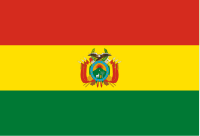## Colombia / Ecuador## India / Niger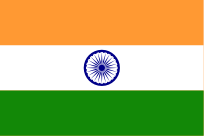## Ireland / Côte d’Ivoire## El Salvador / Nicaragua / Honduras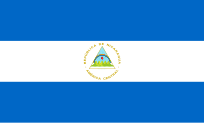## Egypt / Iraq / Syria / Yemen## Luxembourg / The Netherlands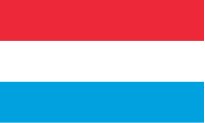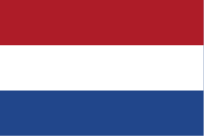## Indonesia / Monaco## Emoji

Each flag has an emoji, so here are the groupings above using emoji icons

• 🇹🇩 🇷🇴
• 🇧🇴 🇬🇭
• 🇨🇴 🇪🇨
• 🇮🇳 🇳🇪
• 🇮🇪 🇨🇮
• 🇸🇻 🇳🇮 🇭🇳
• 🇪🇬 🇮🇶 🇸🇾 🇾🇪
• 🇱🇺 🇳🇱
• 🇦🇩 🇲🇩
• 🇮🇩 🇲🇨

## Related posts

 The groupings are based on Mathematica’s output, but I did some editing. Strictly following Mathematica’s descriptions would have been complicated. For example, Mathematica’s description might say A is similar to B, but not say B is similar to A. Or it might cluster four flags together that could better be split into two pairs.

# Costas arrays

The famous n queens problem is to find a way to position n queens on a n×n chessboard so that no queen attacks any other. That is, no two queens can be in the same row, the same column, or on the same diagonal. Here’s an example solution: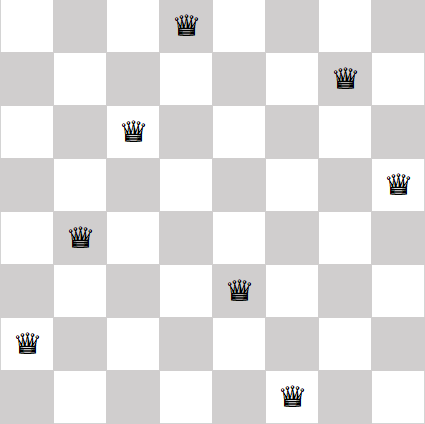## Costas arrays

In this post we’re going to look at a similar problem, weakening one requirement and adding another. First we’re going to remove the requirement that no two pieces can be on the same diagonal. This turns the n queens problem into the n rooks problem because rooks cannot move diagonally.

Next, imagine running stiff wires from every rook to every other rook. We will require that no two wires have the same length and run in the same direction. in more mathematical terms, we require that the displacement vectors between all the rooks are unique.

A solution to this problem is called a Costas array.  In 1965, J. P. Costas invented what are now called Costas arrays to solve a problem in radar signal processing.

Why do we call a solution a Costas array rather than a Costas matrix? Because a matrix solution can be described by recording for each column the row number of the occupied cell. For example, we could describe the eight queens solution above as

(2, 4, 6, 8, 3, 1, 7, 5).

Here I’m numbering rows from the bottom up, like points in the first quadrant, rather than top to bottom as one does with matrices.

Note that the solution to the eight queens problem above is not a Costas array because some of the displacement vectors between queens are the same. For example, if we number queens from left to right, the displacement vectors between the first and second queens is the same as the displacement vector between the second and third queens.

## Visualizing a Costas array

Here’s a visualization of a Costas array of order 5.It’s clear from the plot that no two dots are in the same row or column, but it’s not obvious that all the connecting lines are different. To see that the lines are different, we move all the tails of the connecting lines to the origin, keeping their length and direction the same.There are 10 colored lines in the first plot, but at first you may only see 9 in the second plot. That’s because two of the lines have the same direction but different length. If you look closely, you’ll see that there’s a short purple line on top of the long red line. That is, one line runs from the origin to (1, -1) and another from the origin to (4, -4).

Here is a visualization of a Costas array of order 9.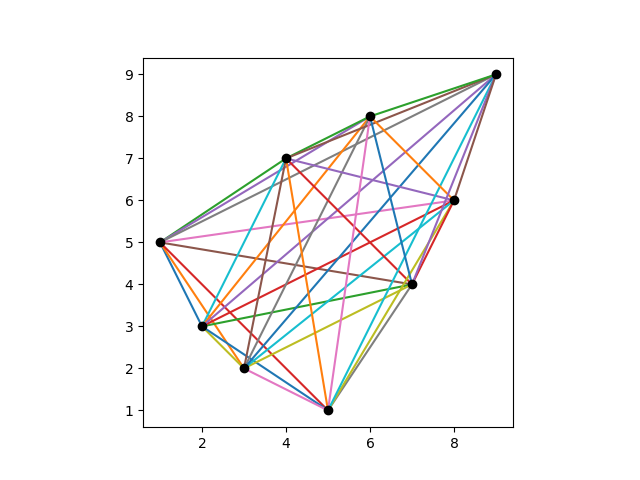And here are its displacement vectors translated to the origin.Here is a visualization of a Costas array of order 15.And here are its displacement vectors translated to the origin.## Generating Costas arrays

There are algorithms for generating some Costas arrays, but not all. Every known algorithm  leaves out some solutions, and it is not know whether Costas arrays exist for some values of n.

The Costas arrays above were generated using the Lempel construction algorithm. Given a prime p  and a primitive root mod p , the following Python code will generate a Costas array of order p – 2.

```
p = 11 # prime
a = 2 # primitive element

# verify a is a primitive element mod p
s = {a**i % p for i in range(p)}
assert( len(s) == p-1 )

n = p-2
dots = []

for i in range(1, n+1):
for j in range(1, n+1):
if (pow(a, i, p) + pow(a, j, p)) % p == 1:
dots.append((i,j))
break
```

## Related posts

 Strictly speaking, no scalable algorithm will enumerate all Costas arrays. You could enumerate all permutation matrices of order n and test whether each is a Costas array, but this requires generating and testing n! matrices and so is completely impractical for moderately large n.

 More generally, the Lempel algorithm can generate a solution of order q-2 where q is a prime power. The code above only works for primes, not prime powers. For prime powers, you have to work with finite fields of order q and the code would be more complicated.

 A primitive root for a finite field of order q is a generator of the multiplicative group of the field, i.e. an element x such that every non-zero element of the field is some power of x.

# Balanced tournament designs

Suppose you have an even number of teams that you’d like to schedule in a Round Robin tournament. This means each team plays every other team exactly once.

Denote the number of teams as 2n. You’d like each team to play in each round, so you need n locations for the games to be played.

Each game will choose 2 teams from the set of 2n teams, so the number of games will be n(2n – 1). And this means you’ll need 2n – 1 rounds. A tournament design will be a grid with n rows, one for each location, and 2n-1 columns, one for each round.

Let’s pause there for just a second and make sure this is possible. We can certainly assign n(2n – 1) games to a grid of size n by 2n-1. But can we do it in such a way that no team needs to be in two locations at the same time? It turns out we can.

Now we’d like to introduce one more requirement: we’d like to balance the games over the locations. By that we mean we’d like a team to play no more than twice in the same location. A team has to play at least once in each location, but not every team can play twice in each location. If every team played once in each location, we’d have n² games, and if every team played twice in each location we’d have 2n² games, but

n² < n(2n – 1) < 2n²

for n greater than 1. If n = 1, this is all trivial, because in that case we would have two teams. They simply play each other and that’s the tournament!

We can’t have each team play exactly the same number of times in each location, but can we have each team play either once or twice in each location? If so, we call that a balanced tournament design.

Now the question becomes for which values of n can we have a balanced tournament design involving 2n teams. This is called a BTD(n) design.

We said above that this is possible if n = 1: two teams just play each other somewhere. For n = 2, it is not possible to have a balanced tournament design: some team will have to play all three games in the same location.

It is possible to have a balanced tournament design for n = 3. Here’s a solution: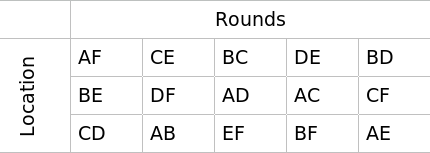In fact this is the solution aside from relabeling the teams. That is, given any balanced tournament design involving six teams, there is a way to label the teams so that you have the design above. Said another way, there is one design in BTD(3) up to isomorphism.

There are balanced tournament designs for all positive n except for n = 2. And in general there are a lot of them. There are 47 designs in BTD(4) up to isomorphism .

## Related posts

 The CRC Handbook of Combinatorial Designs. Colbourn and Dinitz editors. CRC Press, 1996.

# Graphing Japanese Prefectures

The two previous posts looked at adjacency networks. The first used examples of US states and Texas counties. The second post made suggestions for using these networks in a classroom. This post is a continuation of the previous post using examples from Japan.

Japan is divided into 8 regions and 47 prefectures. Here is a network diagram of the prefectures in the Kanto region showing which regions border each other. (In this post, “border” will be regions share a large enough border that I was able to see the border region on the map I was using. Some regions may share a very small border that I left out.)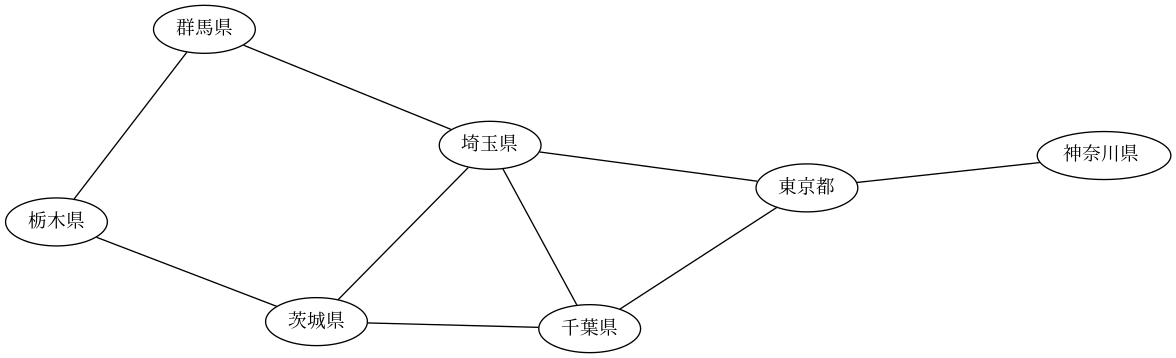This is a good example of why it is convenient in GraphViz to use variable names that are different from labels. I created my graphs using English versions of prefecture names, and checked my work using the English names. Then after debugging my work I changed the label names (but not the connectivity data) to use Japanese names.

To show what this looks like, my GraphViz started out like this

```    graph G {
layout=sfdp
AI [label="Aichi"]
AK [label="Akita"]
AO [label="Aomori"]
...
AO -- AK
AO -- IW
AK -- IW
...
```

and ended up like this

```    graph G {
layout=sfdp
AI [label="愛知県"]
AK [label="秋田県"]
AO [label="青森県"]
...
AO -- AK
AO -- IW
AK -- IW
...
```

Here’s a graph only showing which prefectures border each other within a region.This image is an SVG, so you can rescale it without losing any resolution. Here’s the same image as a PDF.

Because this network is effectively several small networks, it would be easy to look at a map and figure out which nodes correspond to which prefectures. (It would be even easier if you could read the labels!)

Note that there are two islands—literal islands, as well as figurative islands in the image above—Hokkaido, which is its own region, and Okinawa, which a prefecture in the Kyushu region.

Here’s the graph with all bordering relations, including across regions.The image above is also an SVG. And here’s the same image as a PDF.

# Classroom exercise with networks

In the previous post I looked at graphs created from representing geographic regions with nodes and connecting nodes with edges if the corresponding regions share a border.

It’s an interesting exercise to recover the geographic regions from the network. For example, take a look at the graph for the continental United States.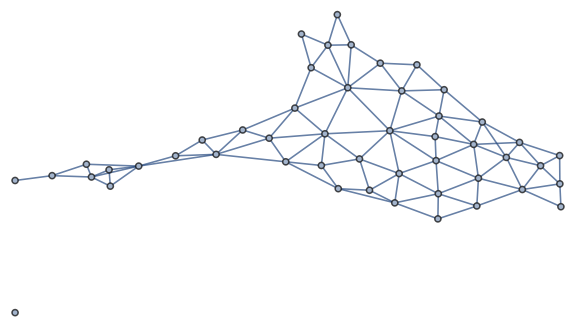It’s easy to identify Alaska in the graph. The node on the left represents Maine because Maine is the only state to border exactly one other state. From there you can bootstrap your way to identifying the rest of the states.

## Math class

This could make a fun classroom exercise in a math class. Students will naturally come up with the idea of the degree of a node, the number of edges that meet that node, because that’s a handy way to solve the puzzle: the only possibilities for a node of degree n are states that border n other states.

This also illustrates that networks preserve topology, not geometry. That is, the connectivity information is retained, but the shape is dramatically different.

## Geography class

Someone asked me on Twitter to make a corresponding graph for Brazil. Mathematica, or at least my version of Mathematica, doesn’t have data on Brazilian states, so I made an adjacency graph using GraphViz.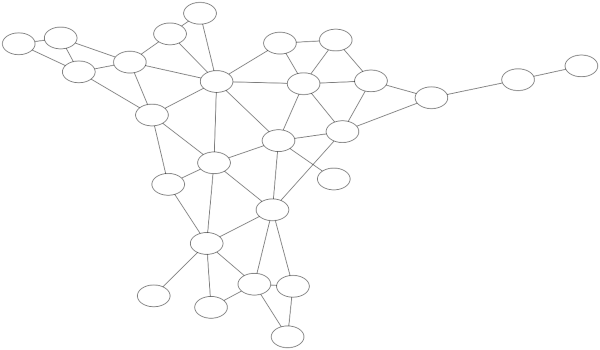Labeling the blank nodes is much easier for Brazil than for the US because Brazil has about half as many states, and the topology of the graph gives you more to work with. Three nodes connect to only one other node, for example.

Here the exercise doesn’t involve as much logic, but the geography is less familiar, unless of course you’re more familiar with Brazil than the US. Labeling the graph will require staring at a map of Brazil and you might accidentally learn a little about Brazil.

## GraphViz

The labeled version of the graph above is available here. And here are the GraphViz source files that make the labeled and unlabeled versions.

The layout of a GraphViz file is very simple. The file looks like this:

```    graph G {

layout=sfdp

AC [label="Acre"]
AL [label="Alagoas"]
...
AC -- AM
AC -- RO
...
}
```

There are three parts: a layout, node labels, and connections.

GraphViz has several layout engines, and the `sfdp` one matched what I was looking for in this case. Other layout options lead to overlapping edges that were confusing.

The node names AC, AL, etc. do not appear in the output. They’re just variable names for your convenience. The text inside the label is what appears in the final output. I’ll give an example in the next post in which it’s very convenient for the variables to be different from the labels. The order of the labels doesn’t matter, only which variables are associated with which labels.

Finally, the lines with variables separated by dashes are the connection data. Here we’re telling GraphViz to connect node AC to nodes AM and RO. The order of these lines doesn’t matter.